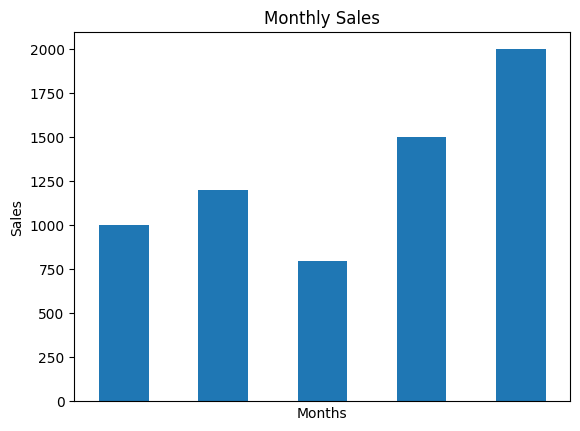To remove Xticks from a plot in Pandas or Matplotlib we have multiple options.

## Remove Xticks in Matplotlib

• turn off all axis

• `plt.axis('off')`
• remove with tick_params

``````plt.tick_params(\
axis='x',          # changes apply to the x-axis
which='both',      # both major and minor ticks are affected
bottom='off',      # ticks along the bottom edge are off
top='off',         # ticks along the top edge are off
labelbottom='off') # labels along the bottom edge are off
``````
• set empty ticks
``````plt.xticks([], [])
ax.set_xticks([])
ax.set_xticks([], minor=True)
``````

## Steps to plot 2 variables

• Import libraries pandas and matplotlib
• Create a sample DataFrame
• Create a bar plot
• Remove Xticks
• Set axis labels
• Show the plot

Months Sales
0 Jan 1000
1 Feb 1200
2 Mar 800
3 Apr 1500
4 May 2000

## Example

``````import pandas as pd
import matplotlib.pyplot as plt

# Create a sample DataFrame
data = {'Months': ['Jan', 'Feb', 'Mar', 'Apr', 'May'],
'Sales': [1000, 1200, 800, 1500, 2000]}
df = pd.DataFrame(data)

# Create a bar plot
ax = df.plot(x='Months', y='Sales', kind='bar', legend=False)

# Remove Xticks
ax.set_xticks([])

# Set axis labels
plt.xlabel('Months')
plt.ylabel('Sales')

# Set the plot title
plt.title('Monthly Sales')

# Show the plot
plt.show()
``````

## Output